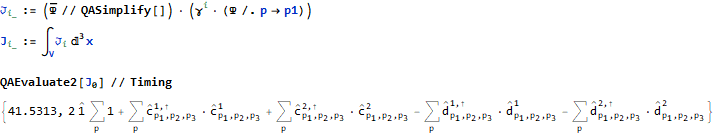### Article Index

The following is an extension of the Mathematica application Quantum Algebra.

The extended version of the application Quantum Algebra can be found here.

## The Problem

In my research on quantum physics, I also study the book "Student Friendly Quantum Field Theory" by Robert D. Klauber. This led to the question or idea of ​​whether some of the calculations listed could not be facilitated by Mathematica.

As a Mathematica package to support the calculations, Quantum Algebra seemed to me to be suitable - at least somewhat. But some of the mathematical symbols used in the book were not supported by Quantum Algebra. Quantum algebra had to be extended. So an extended application resulted. This extension and what it does should be presented below.

Essentially these are summation, integration and derivation symbols, the operator notation already present in the basic package and the support of matrices. All are supported by appropriate rules for simplification and reshaping - just as you would do manually.

## Notations

The Quantum Algebra extension uses a number of notations to improve the readability of expressions. They supplement the notations that already exist in the basic package. This also takes into account the need for documentation.

Notations of the basic package:

• Operator Notation
• Superdagger
• Centerdot

• Integral
• Total Notation
• Partial Derivative
• Small Circle

### Overview

 Name Sample Notation FullForm - Simplified or Complete Expression Operator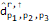`Operator[d, "H", `` List[Subscript[p, 1], Subscript[p, 2], Subscript[p, 3]], List]` SuperDagger`Hermitian[Operator[d, `` List[Subscript[p, 1], Subscript[p, 2], Subscript[p, 3]], List]]` CenterDot`NonCommutativeMultiply[Operator[d,"H",List[Subscript[p, 1]], List],``Operator[d, List[Subscript[p, 1]], List]]` QAIntegrate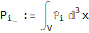`QAIntegrate[Subscript[p, i], V, 3, x]` QASum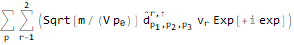`QASum[Operator[d, "H",  List[Subscript[p, 1], Subscript[p, 2], Subscript[p, 3]], List],p]` QAD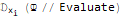`QAD[\[psi] // Evaluate, Subscript[x, i]]` SmallCircle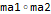` SmallCircle[ma1, ma2]`

## Syntax and Semantics

In the following, the notations, their associated FullForm constructs and the semantics are shown.

1.  Operator
1. An Operator has the following Full Form: `Operator[Name, "H", { Lower Indices }, { Upper Indices }]`
The lists of upper and lower indices may also be empty, the indicator for an adjoint operator "H" may be missing.
2. Sample notation
See Notations, Operator, Sample notation
3. Semantics
Name indicates the name of the operator, "H" indicates the associated adjoint operator, the two lists contain the lower and upper indices of the notation. The addition to Quantum Algebra often makes use of the convention that the lower indices include wavenumber or momentum variables in subscript representation. The 3 - momentum would consist of the indices p1, p2, p3 and would be supplemented by p0 as energy for the 4 - momentum.
2. Hermitian
1. Full Form Syntax
The Hermitian function determines the adjoint operator for an operator, operator expression or an operator matrix:
`op2 = Hermitian[op1];`
2. Sample Notation
The super-dagger symbol on an expression can either indicate an adjoint operator or cause the generation of the adjoint of a complete expression, see e.g. Klein - Gordon - Equation a.
3. Semantics
An adjoint operator is a mirror operator for a dual space (see literature on quantum mechanics).
3. NonCommutativeMultiply
1. Full Form Syntax
Operators in quantum mechanics are generally noncommutative. Their multiplication therefore requires an extra symbol:
`NonCommutativeMultiply[op1, op2]` or `op1 ** op2` or use the shortcut icon CenterDot.
2. Sample Notations
See e.g. Notations, CenterDot.
3. Semantics
4. QAIntegrate
1. Full Form Syntax
`QAIntegrate[Integrand, Area, Dim, Var(s)]`
2. Sample Notation
See e.g. Notations, QAIntegrate.
3. Semantics
This is an integral over a generally multidimensional area Area. Dim is the dimension and Var is an integration variable. You can also specify more than one, with the associated notation supporting a maximum of 2. The range may either be finite - a symbol (e.g., V) is given - or infinity - here the infinity symbol is given.
5. QASum
1. Full Form Syntax
The syntax is similar to integration, with no scope or dimension. In the end, it is always summed from -Infinity to + Infinity, and the dimensionality is assumed to be 3.
`QASum[Expression, Var(s)] `
2. Sample Notation
See e.g. Notations, QASum.
3. Semantics
This is a summation over an expression, the 3-dimensional variable Var runs over all integer values. You can also specify 2 of them or the expression will be simplified accordingly.
1. Full Form Syntax
`QAD[Quexp, Var]`
2. Sample Notation
3. Semantics
This is a derivative of quantum expressions Quexp to variables Var.

## Implementation

The rules for making calculations and simplifications can be divided into different categories:

1. Automatic rules
These are applied automatically after initialization and usually result in a simplification of the expression.
2. "Replacement rules"
There are predefined and freely definable (application specific) substitution rules. A set of such rules is generally summarized under a name. The reason that they are not automatically executed is that the benefits are typically situational. So it may be desirable that sums are summarized or even divided, because one or the other leads to a simplification.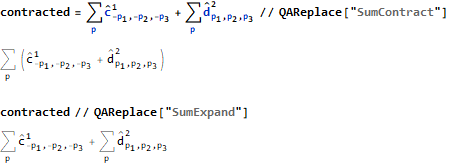3. Assumptions
The Mathematica function Simplify can perform simplifications based on certain assumptions. To make this more manageable, sets of assumptions can be grouped under one name. They can then be referenced under this name.
For example: `exp // QASimplify["Dirac"] `
The expression exp is simplified here on the assumptions of the name "Dirac".
Assumptions are in general application-specific.

## Unit Tests

The Quantum Algebra supplement is accompanied by a series of unit tests. They are intended to ensure that changes to the code remain executable.

At the same time, it helps to track which rules lead to which simplifications.

``````(* Combine product of Integrations *)
Test[
QAIntegrate[Subscript[x, 1], V, 3, x] QAIntegrate[Subscript[y, 2], V, 3, y],
QAIntegrate[Subscript[x, 1] Subscript[y, 2], V, 3, x, y],
TestID->"Integrate-20181213-P3R4W5"
]

(* Apply DiracDelta in Integrate about 2 integration ranges *)
Test[
QAIntegrate[
Subscript[x, 1] Subscript[y, 2] *
DiracDelta[Subscript[x, 1] - Subscript[y, 1], Subscript[x, 2] - Subscript[y, 2], Subscript[x, 3] - Subscript[y, 3]],
V, 3, x, y],
QAIntegrate[Subscript[x, 1] Subscript[x, 2], V, 3, x],
TestID->"Integrate-20181213-N8K6H2"
]

(* Integrate about Exponential resolved to DiracDelta *)
Test[
QAIntegrate[
Power[E, I (Subscript[k, 1] (Subscript[x, 1] - Subscript[y, 1]) + Subscript[k, 2] (Subscript[x, 2] - Subscript[y, 2]))],
Infinity, 2, k],
DiracDelta[Subscript[x, 1] - Subscript[y, 1], Subscript[x, 2] - Subscript[y, 2]] 4 Pi^2,
TestID->"Integrate-20181216-P1R1T9"
]

(* Test of Name Ordering Rule, op1 ** op2 are in reverse order *)
Test[
(op1 = Operator[q, "H", { Subscript[k, 1], Subscript[k, 2] }, {}];
op2 = Operator[p, "H", { Subscript[l, 1], Subscript[l, 2] }, {}];
(op1 ** op2) /. { DefineNameOrderingRule[False, 2] }),
op2 ** op1,
TestID->"NameOrdering-20181226-X4S4F9"
]

(* Derivation of an Operator Product *)
Test[
QAD[Operator[a, { Subscript[x, 1] }, {}] ** Operator[b, { Subscript[x, 1] }, { s }], Subscript[x, 1]],

QAD[Operator[a, { Subscript[x, 1] }, {}], Subscript[x, 1]] ** Operator[b, { Subscript[x, 1] }, { s }] +
Operator[a, { Subscript[x, 1] }, {}] ** QAD[Operator[b, { Subscript[x, 1] }, { s }], Subscript[x, 1]],

TestID->"Derivation-20181217-L0J8L0"
]
``````

## Sample Applications

The sample applications are given in the form of notebooks. For the time being there are the following:

• A notebook for the Klein - Gordon - equation
• A notebook for the Dirac - equation

In addition to local rules, they contain a series of calculations, e.g. Hamilton - operator, 3 - momentum and evaluation of charge.

### Klein - Gordon - Equation

In all cases, the free fields that solve the field equations are specified.

1. The commutator of the fields, when applying the commutation relations for the coefficients of the fields, leads to the commutation relations of the fields themselves.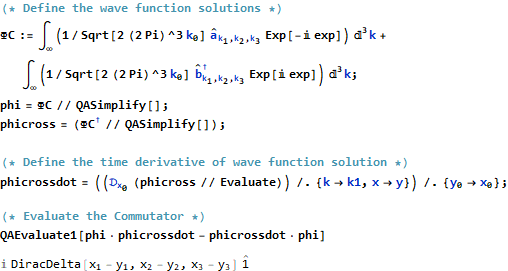2. The Hamiltonian as an expression dependent on the fields leads to an expression in the coefficients of the fields (creation and annihilation operators).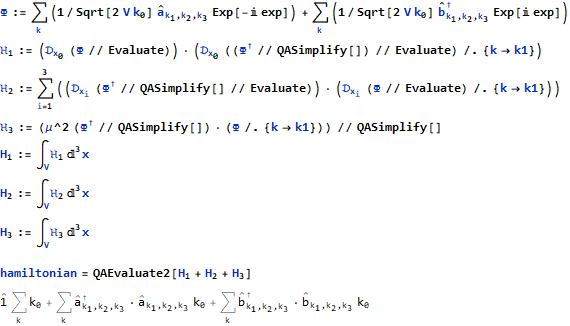3. The same applies to the expression of the 3-momentum.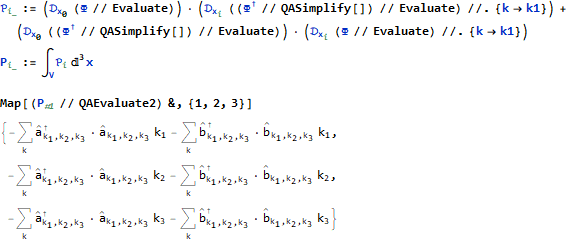4. The same applies to the expression for the charge, which results from a continuity equation.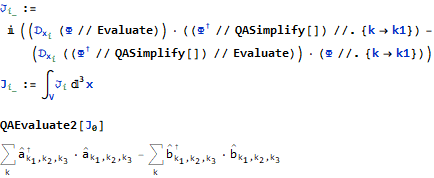## Sample Applications - Continuation

### Dirac - Equation

The free fields that solve the field equations are again specified: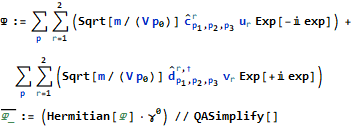1. The Hamilton operator is defined and evaluated: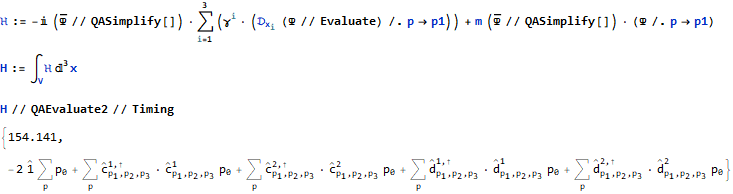2. The 3 - momentum is defined and evaluated: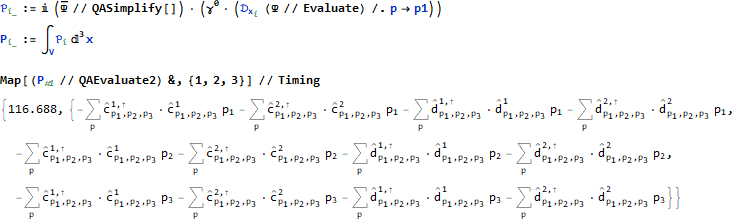3. The 4 - current is defined and the charge is evaluated (without multiplicative charge constant):